Unconstrained clustering analysis is a strategy for intra-site spatial grouping of artifact data first presented by Whallon (1984) with later modifications suggested by Kintigh (1990). The goal of this approach is to partition a site into areas with respect to the relative proportions of artifact categories found in specific locations. The approach can be conducted on point located data but, importantly for many archaeological datasets, also on gridded data (which is sometimes preferable). The following example applies the grid-based unconstrained clustering approach to a dataset from Pincevent Section 36 (see Simek and Larick 1983) demonstrating the step by step procedures in R.

The first step in conducting UC on point plotted data is to read in the data file of XY coordinates and artifact types and then set up a grid based on the extents of those coordinates. This grid can then be plotted with artifacts shown symbolized by category.

# read in the Pincevent data file, columns E=Easting, N=Northing, TYPE=artifact
# category

# define the minima and maxima on the x and y-axes and create a vector of points
# at 1 meter spacing
x <- seq(floor(min(Pincevent$E)), ceiling(max(Pincevent$E)), by = 1)
y <- seq(floor(min(Pincevent$N)), ceiling(max(Pincevent$N)), by = 1)
# convert these two vectors into an xy grid
grid <- expand.grid(x, y)

# set up an empty plot - option 'n'
plot(grid, t = "n", xlab = "X", ylab = "Y")
abline(v = x, h = y, lwd = 0.5, col = "lightgray")  #plot grid lines
# plot artifacts with symbols representing types
points(Pincevent$E, Pincevent$N, pch = Pincevent$TYPE + 1, cex = 0.5)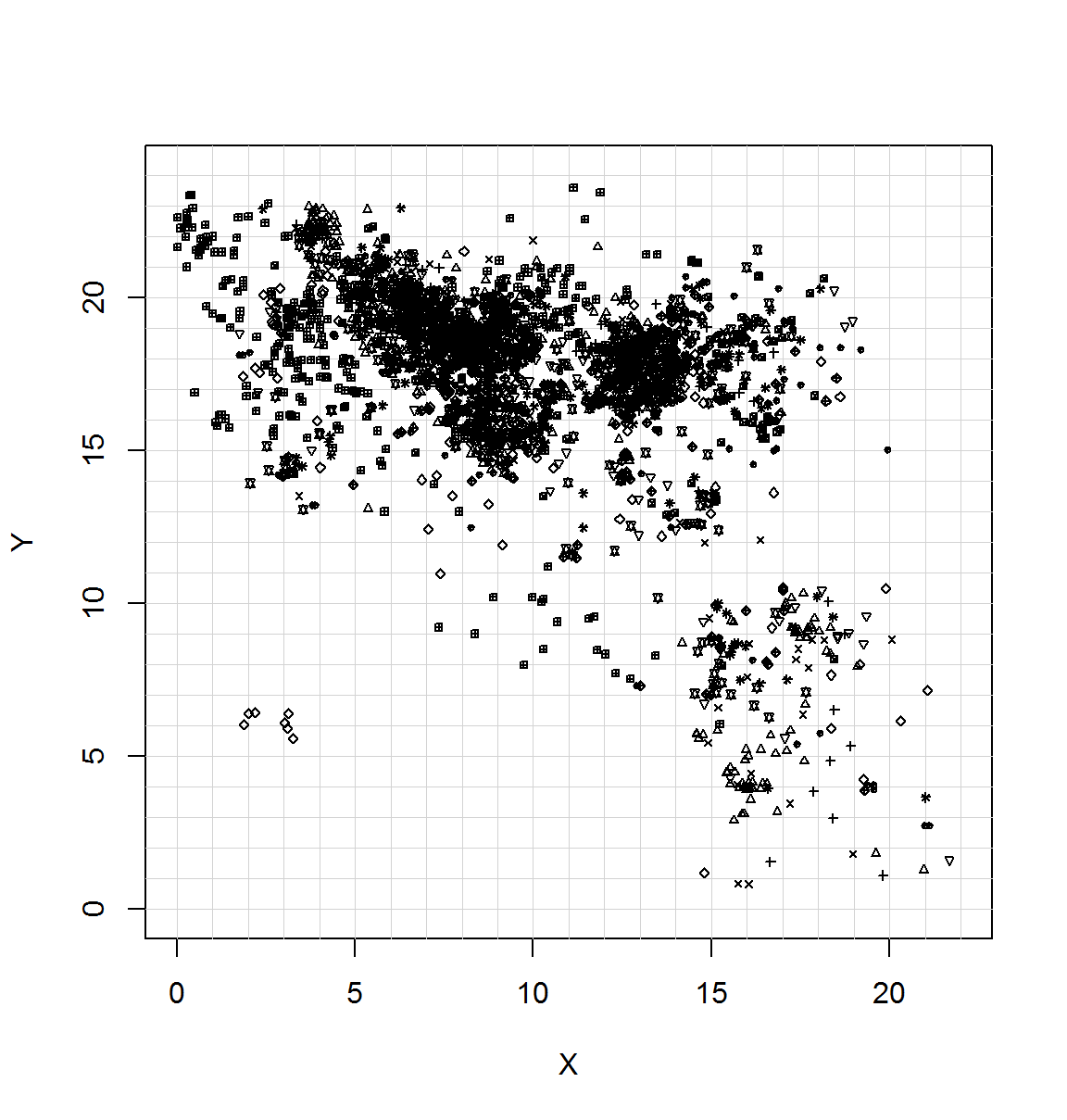The next step is to assign the point plotted data to individual grid squares. This is done using the findInterval command. The results are then pooled in a table and displayed. # create data frame with point plotted artifacts assigned to grid squares binxy <- data.frame(x = findInterval(Pincevent$E, x) + (min(x) - 1), y = findInterval(Pincevent$N, y) + (min(y) - 1)) (results <- table(binxy)) #pool results in table and display ## y ## x 0 1 2 3 4 5 6 7 8 9 10 11 12 13 14 15 16 ## 0 0 0 0 0 0 0 0 0 0 0 0 0 0 0 0 0 1 ## 1 0 0 0 0 0 0 1 0 0 0 0 0 0 0 0 3 4 ## 2 0 0 0 0 0 0 2 0 0 0 0 0 0 1 4 4 6 ## 3 0 0 0 0 0 2 2 0 0 0 0 0 0 4 12 2 6 ## 4 0 0 0 0 0 0 0 0 0 0 0 0 0 1 2 7 7 ## 5 0 0 0 0 0 0 0 0 0 0 0 0 1 1 3 2 7 ## 6 0 0 0 0 0 0 0 0 0 0 0 0 0 0 2 4 6 ## 7 0 0 0 0 0 0 0 0 0 1 1 0 2 2 2 16 30 ## 8 0 0 0 0 0 0 0 0 1 0 1 0 1 2 14 63 46 ## 9 0 0 0 0 0 0 0 1 0 0 1 1 0 0 16 49 24 ## 10 0 0 0 0 0 0 0 0 1 1 2 3 0 3 7 16 24 ## 11 0 0 0 0 0 0 0 0 1 2 0 4 1 1 0 2 24 ## 12 0 0 0 0 0 0 0 4 1 0 0 1 3 3 16 6 47 ## 13 0 0 0 0 0 0 0 0 1 0 1 0 5 4 3 4 41 ## 14 0 1 0 0 0 6 1 3 3 2 0 1 8 7 2 2 7 ## 15 1 0 1 8 9 2 2 8 16 7 0 0 1 6 0 3 12 ## 16 1 1 0 10 6 3 2 4 4 3 1 0 1 1 2 14 11 ## 17 0 0 0 2 1 4 2 3 7 15 5 0 0 0 0 0 1 ## 18 0 1 1 0 1 3 1 1 7 4 2 0 0 0 0 0 2 ## 19 0 2 0 2 3 0 0 1 2 1 1 0 0 0 0 1 0 ## 20 0 1 1 0 0 0 1 0 1 0 0 0 0 0 0 0 0 ## 21 0 1 1 1 0 0 0 1 0 0 0 0 0 0 0 0 0 ## y ## x 17 18 19 20 21 22 23 ## 0 0 0 1 1 12 11 2 ## 1 2 3 6 6 6 1 0 ## 2 6 8 12 4 1 3 1 ## 3 11 11 34 6 16 32 0 ## 4 13 9 13 19 18 23 0 ## 5 8 27 40 51 18 3 0 ## 6 30 47 117 57 8 1 0 ## 7 43 95 87 17 2 0 0 ## 8 50 107 52 8 2 0 0 ## 9 27 48 49 15 2 1 0 ## 10 16 13 14 17 1 0 0 ## 11 18 19 11 4 1 1 2 ## 12 108 91 23 2 0 0 0 ## 13 78 85 13 1 2 0 0 ## 14 27 17 17 8 4 0 0 ## 15 11 28 2 2 0 0 0 ## 16 5 12 10 3 1 0 0 ## 17 2 5 4 1 0 0 0 ## 18 2 2 2 3 0 0 0 ## 19 0 1 0 0 0 0 0 ## 20 0 0 0 0 0 0 0 ## 21 0 0 0 0 0 0 0 Next, I sum artifact totals by grid square and display the result on the original map (with 0 cells removed). d <- as.data.frame.table(results) xx <- x[-length(x)] + 0.5 * diff(x) d$x <- xx[d$x] yy <- y[-length(y)] + 0.5 * diff(y) d$y <- yy[d$y] plot(grid, t = "n", xlab = "X", ylab = "Y") abline(v = x, h = y, lwd = 0.5, col = "lightgray") with(d[which(d[, 3] > 0), ], text(x, y, label = Freq, cex = 0.5))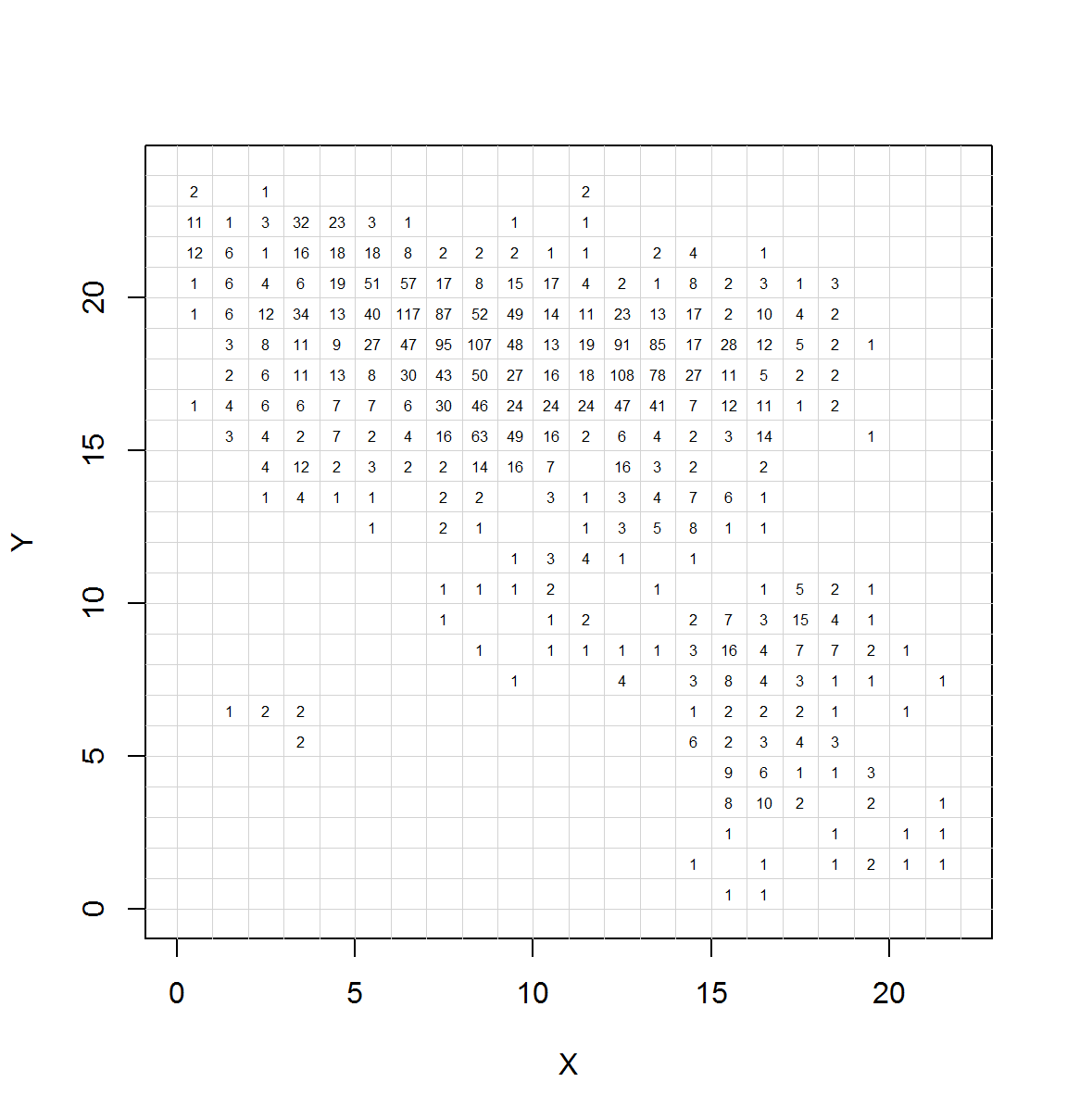Following this, I then create a matrix of the frequencies of each artifact category in each excavated grid square and then calculate row percents. In this example, I remove any grid square with fewer than 5 artifacts. # create object of grid squares and type occurrence grid.type <- as.data.frame(cbind(paste(binxy$x, binxy$y), Pincevent$TYPE))
# pool list into a data frame matrix
grid.tab <- as.data.frame.matrix(table(grid.type))
# create matrix from table excluding grids with fewer than 5 artifacts
grid.ct <- grid.tab[which(rowSums(grid.tab) >= 5), ]
# calculate row percents of the above matrix
grid.p <- prop.table(as.matrix(grid.ct), margin = 1) * 100

Next, I conduct k-means cluster analysis on the matrix of artifact percentages by grid square. In order to choose an appropriate number of clusters, I conduct a randomization procedure to assess changes in the global Sum of Squared Error (SSE) for varying cluster levels in both the original data and in 1000 column randomized versions of those data. The results are then plotted both in terms of absolute SSE and in terms of the difference between the actual and mean randomized data.The goal of these plots is to identify any inflection points in SSE values as well as the pattern of departures from random at varying cluster levels (see Kintigh 1990 for more details).

# define function for calculating within group sum of squares for kmeans
# solutions 1 through max K
kmeans.wss <- function(data, maxk) {
wss <- (nrow(data) - 1) * sum(apply(data, 2, var))
for (i in 2:maxk) wss[i] <- sum(kmeans(data, centers = i, iter.max = 100, nstart = 10)$tot.withinss) return(wss) } set.seed(100) # set seed for repeatability # calculate wss for cluster levels 1 through 15 for the original data kmeans.orig <- kmeans.wss(grid.p, maxk = 15) # the following chunk creates 1000 column randomized version of the original # dataset calculates global SSE for cluster solutions 1 through 15 rand.out <- NULL nsim <- 1000 for (i in 1:nsim) { rand.tab <- apply(grid.p, 2, sample) if (min(rowSums(rand.tab) > 0)) { rand.out <- rbind(rand.out, kmeans.wss(rand.tab, maxk = 15)) } } # next I plot the SSE scores by cluster solution for the original and the mean of # the randomized data plot(kmeans.orig, type = "b", col = "blue", xlab = "Number of Clusters", ylab = "Sum of Squares") points(colMeans(rand.out), type = "l", col = "red", lty = 2) legend("topright", c("Original Data", "Mean of 1000 Random Runs"), col = c("blue", "red"), lty = c(1, 2))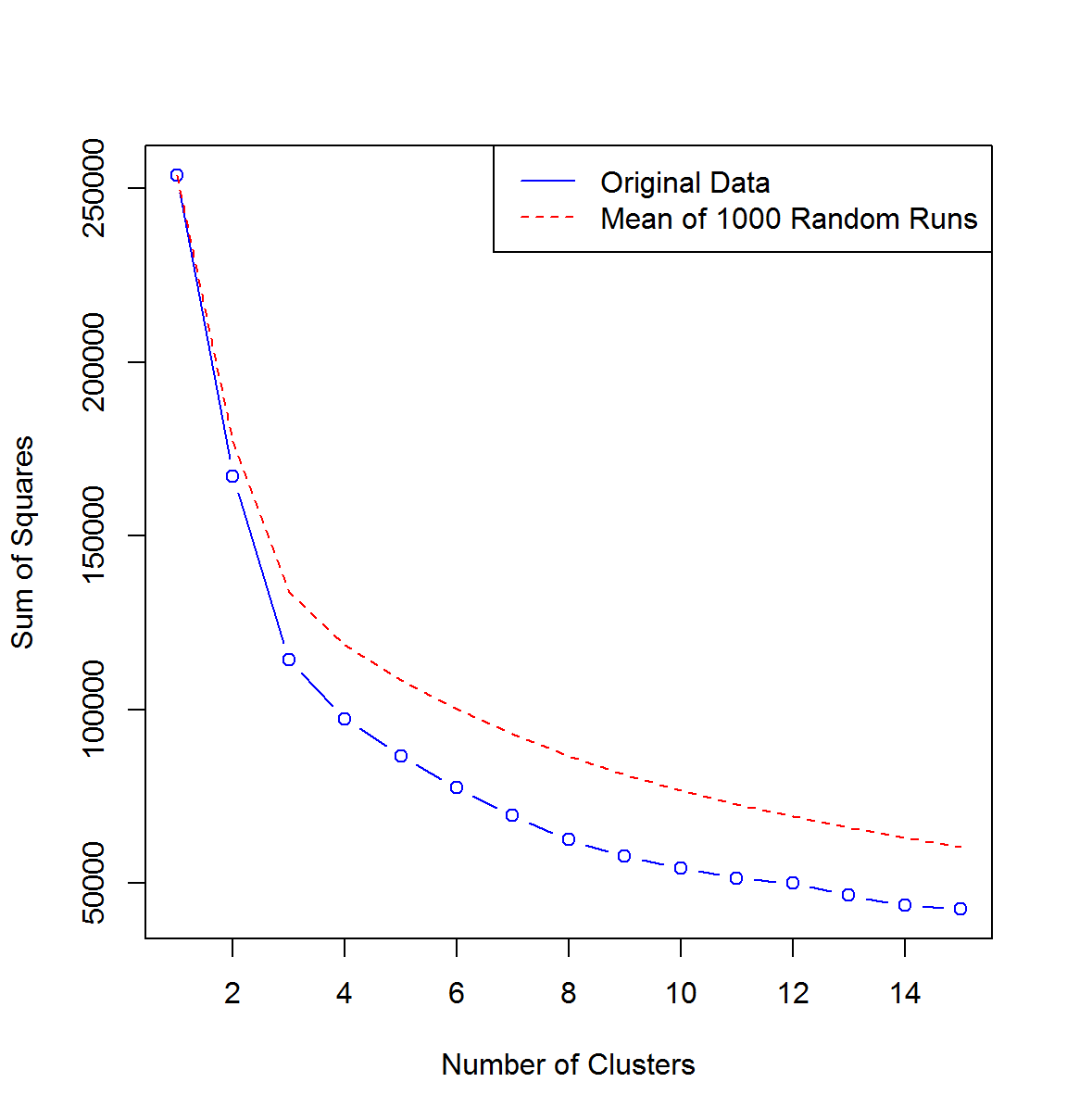# finally, I plot the difference between the mean random SSE and the actual SSE # from the original data plot(colMeans(rand.out) - kmeans.orig, type = "b", col = "blue", xlab = "Number of Clusters", ylab = "Actual SSE - Mean Randomized SSE")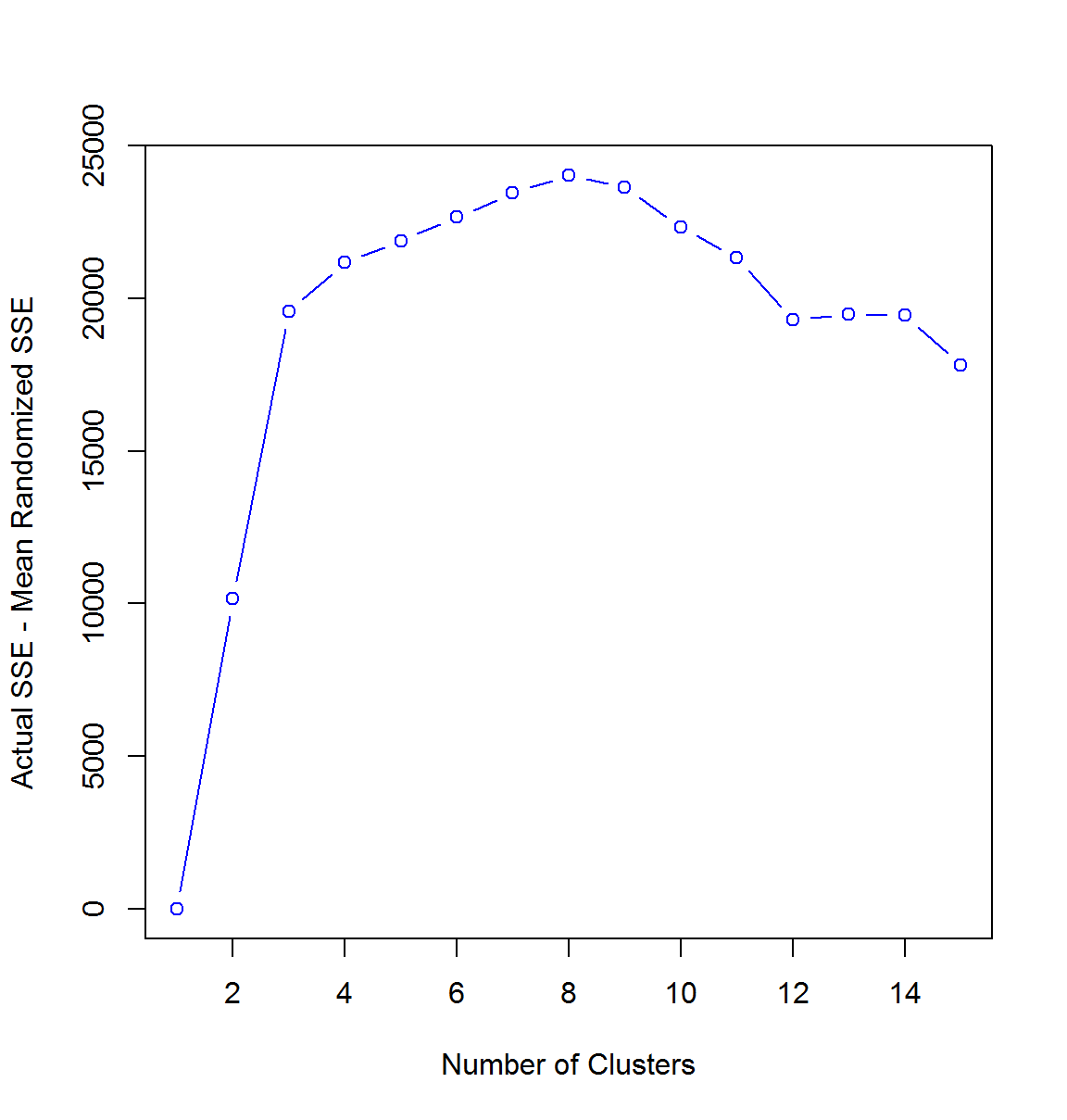Based on the plots displayed above, I select the 8 cluster solution for further analysis as this level represents the furthest departure from random. nclust <- 8 # create matrix of cluster assignments by grid for 8 (nclust) cluster solution kmeans.clust <- as.matrix(kmeans(grid.p, nclust, iter.max = 100, nstart = 100)$cluster)
# create matrix of grid locations baed on row name of the previous matrix
grid.k1 <- as.matrix(do.call("rbind", strsplit(as.character(row.names(kmeans.clust)),
" ", fixed = TRUE)))

# define a midpoint of each grid square for plotting
xx <- as.numeric(grid.k1[, 1]) + 0.5
yy <- as.numeric(grid.k1[, 2]) + 0.5

# plot the original map again with cluster assignments displayed in each grid
# square with 5 or more artifacts
plot(grid, t = "n", xlab = "X", ylab = "Y")
abline(v = x, h = y, lwd = 0.5, col = "lightgray")
text(xx, yy, label = kmeans.clust, cex = 0.75)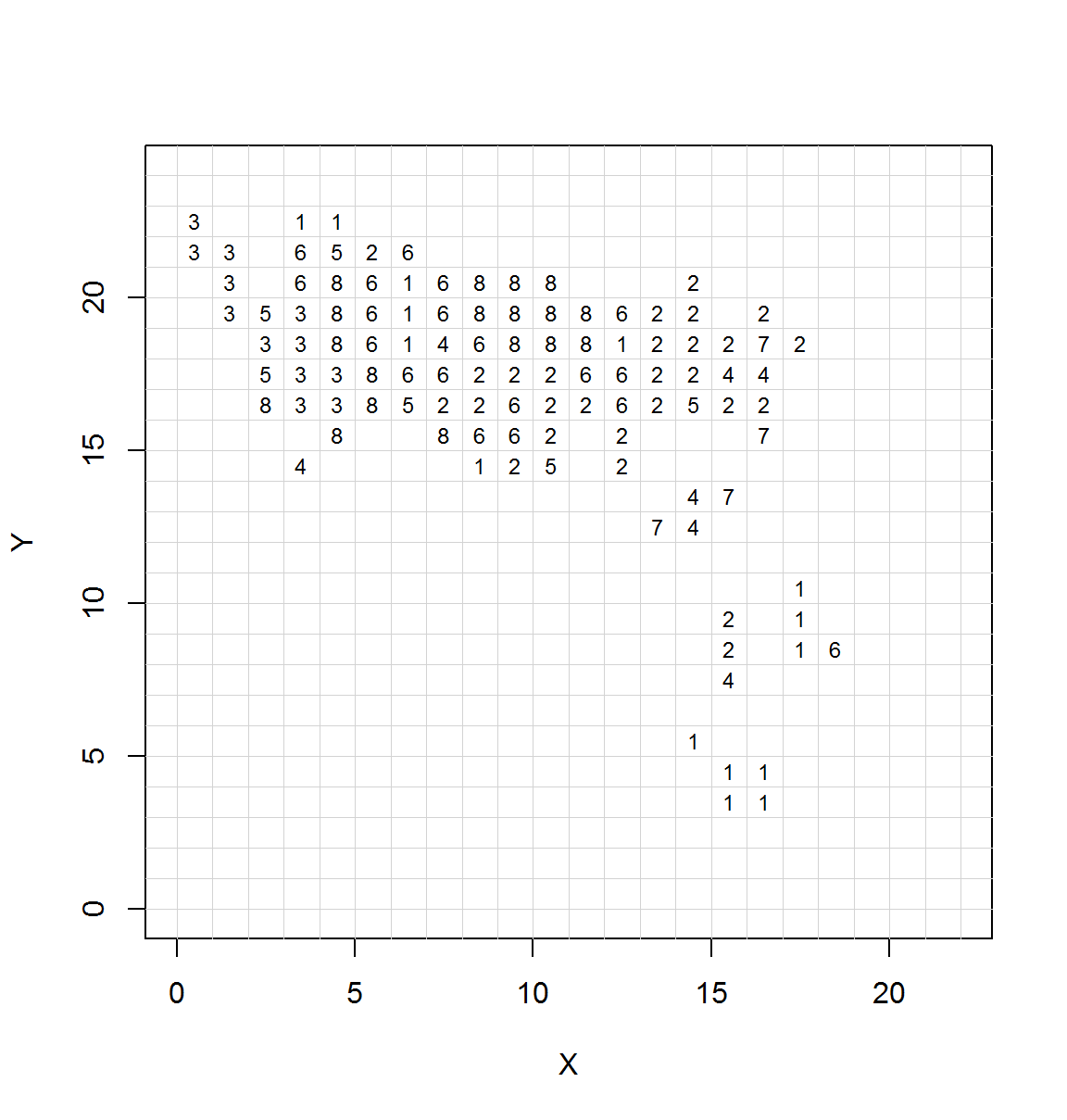The next step is to assess the contiguity of cluster assignments in the map grid. In order to do this, I assign contiguity score for each grid square (>5 artifacts) as the number of adjacent squares that are of the same cluster solution (with grids touching at the diagonal assigned 0.5). Thus the highest level of contiguity a given cluster level can have at one location is 6. This measure is then summed to produce both cluster level and global contiguity scores.

# create matrix of cluster assignments by numeric grid coordinates
clust.mat <- cbind(as.numeric(grid.k1[, 1]), as.numeric(grid.k1[, 2]), kmeans.clust[,
1])

# define function for calculating contiguity as described above (please excuse
# the ugly code)
contig <- function(clust.mat) {
con.score <- cbind(clust.mat, 0)
contig.out <- matrix(0, nclust + 1, 2)  #setup matrix for output
colnames(contig.out) <- c("Count", "Obs Contig")
row.names(contig.out) <- c(seq(1:nclust), "ALL")
# check each contiguous grid square for cluster
for (i in 1:nrow(clust.mat)) {
adj4[1, ] <- c(clust.mat[i, 1] + 1, clust.mat[i, 2], 0)
adj4[2, ] <- c(clust.mat[i, 1] - 1, clust.mat[i, 2], 0)
adj4[3, ] <- c(clust.mat[i, 1], clust.mat[i, 2] + 1, 0)
adj4[4, ] <- c(clust.mat[i, 1], clust.mat[i, 2] - 1, 0)
adj4[5, ] <- c(clust.mat[i, 1] + 1, clust.mat[i, 2] + 1, 0)
adj4[6, ] <- c(clust.mat[i, 1] + 1, clust.mat[i, 2] - 1, 0)
adj4[7, ] <- c(clust.mat[i, 1] - 1, clust.mat[i, 2] - 1, 0)
adj4[8, ] <- c(clust.mat[i, 1] - 1, clust.mat[i, 2] + 1, 0)
for (j in 1:8) {
temp <- which(clust.mat[, 1] == adj4[j, 1] & clust.mat[, 2] == adj4[j,
2])
if (length(temp) > 0) {
}
}
# calculate contiguity score for each grid
3] == clust.mat[i, 3])) * 0.5)
}
# calculate global and cluster level contiguity scores and output
for (m in 1:nclust) {
contig.out[m, 1] <- length(con.score[which(con.score[, 3] == m), 4])
contig.out[m, 2] <- sum(con.score[which(con.score[, 3] == m), 4])
}
contig.out[nclust + 1, 1] <- nrow(con.score)
contig.out[nclust + 1, 2] <- sum(con.score[, 4])
return(contig.out)
}

# display contiguity scores by cluster and for all clusters
(contig.orig <- contig(clust.mat))
##     Count Obs Contig
## 1      15         21
## 2      27         59
## 3      12         30
## 4       7          4
## 5       6          0
## 6      19         25
## 7       4          0
## 8      18         44
## ALL   108        183

In order to have some basis for interpreting the contiguity scores, it is useful to estimate the probability of getting a contiguity score as high or higher than the observed. In order to do this, I conduct contiguity analysis on 1000 randomized datasets by permuting cluster assignments. I then estimate the probability by determining how many random datasets produce contiguity scores greater than or equal to the observed for both the global score and for each cluster.

nsim <- 1000  #set number of random runs

# calculate estimated probability of contiguity by permuting cluster assignments
est.prob <- matrix(0, nclust + 1, 1)
for (i in 1:nsim) {
temp <- which(contig(cbind(clust.mat[, 1:2], sample(clust.mat[, 3])))[, 2] -
contig(clust.mat)[, 2] >= 0)
est.prob[temp, ] <- est.prob[temp, ] + 1
}

# create table of contiguity and estimated probability
contig.prob <- cbind(contig.orig, est.prob/nsim)
colnames(contig.prob) <- "estimated prob"

contig.prob  #display results
##     Count Obs Contig estimated prob
## 1      15         21          0.005
## 2      27         59          0.000
## 3      12         30          0.000
## 4       7          4          0.125
## 5       6          0          1.000
## 6      19         25          0.018
## 7       4          0          1.000
## 8      18         44          0.000
## ALL   108        183          0.000

As the results above show the overall level of global contiguity is highly significantly (p < 0.001). Clusters 2, 3, 4, 6, 8 are also all highly contiguous with very low probabilities that such contiguity could result from chance. Clusters 5 and 7, both of which represent small numbers of grid squares, are highly dispersed. Cluster 1 is somewhat dispersed with an estimated probability of 0.136 indicating that 136 out of 1000 random runs produced contiguity scores greater than or equal to the observed for that cluster.

Now that I have demonstrated non-random clustering overall and for specific clusters, the next step is to look at the compositions of individual clusters.

clust.comp <- matrix(0, nclust, length(unique(Pincevent\$TYPE)))
row.names(clust.comp) <- seq(1:nclust)
for (i in 1:nclust) {
clust.comp[i, ] <- round(prop.table(as.matrix(colSums((grid.ct)[which(kmeans.clust[,
1] == i), ])), 2) * 100, 2)
}
colnames(clust.comp) <- c("Blades", "Ribs", "L. bones", "Awls", "Burins", "Nucleus",
"Scraper", "Antler", "Mand/teeth", "Metacarpals", "Metatarsals")

# reorder columns for ease of interpretation and display results
(clust.comp <- clust.comp[, c(1, 4:11, 2, 3)])
##   Blades Awls Burins Nucleus Scraper Antler Mand/teeth Metacarpals
## 1  67.11 7.38   8.95    0.45    3.80   1.12       1.34        1.79
## 2  11.14 4.07   6.48    5.87    4.22   7.23      17.02       17.62
## 3   3.82 0.00   0.00    0.76    0.00   0.76       1.53        0.00
## 4   5.48 6.85   2.05    2.74    4.79   7.53      15.07        8.90
## 5   8.93 1.79   3.57   41.07    8.93   0.00       7.14        0.00
## 6  32.17 6.23   8.04    4.02    5.84   5.71       4.93        6.23
## 7   2.70 2.70   0.00    8.11    0.00  54.05       5.41        5.41
## 8   8.46 2.42   3.02    3.63    3.63   4.83       8.46        6.95
##   Metatarsals  Ribs L. bones
## 1        1.34  5.37     1.34
## 2       10.39 13.55     2.41
## 3        1.53  3.05    88.55
## 4        4.79 39.73     2.05
## 5        3.57 10.71    14.29
## 6        4.28 14.66     7.91
## 7       16.22  5.41     0.00
## 8        7.55 12.99    38.07

Detailed interpretation of the results presented above will require both substantive knowledge of the materials/cultural context as well as in-depth assessment of the spatial patterning illustrated here. The UC presented above does present a few obvious strong patterns for discussion. First, there is a clear separation of clusters dominated by bones and those dominated by stone tools. Indeed, cluster 8 is largely long bones, perhaps representing a food processing area. Cluster 6 is dominated by backed blades and formal lithic tools perhaps suggesting areas used extensively for cutting and graving activities. Further, cluster 5, though small and non-contiguous, appears to be dominated by nuisance materials such as nuclei and large animals bones (ribs, long bones) perhaps suggesting areas where trash was piled/discarded. Notably, cluster 5 is located around the edges of the areas defined as possible habitation structures by Simek and Larick. As this example illustrates, even when clusters are non-contiguous, they may still be informative.